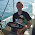## Wednesday, November 9, 2011

### Five Gallons At A Time: Blending, Priming Sugar and Alcohol

Each of the last three years, I brewed a batch of English Ale and set aside a gallon to age on oak chips that were originally inoculated with Flanders Red microorganisms. I blended the remaining four gallons of each batch with the aged beer from the previous year, then added corn sugar to carbonate. If you know the alcohol content of each beer in a blend, you can use the mixing formula to estimate the alcohol content of the blended beer before you add priming sugar:

Blended ABV = (ABV1 x Volume1 + ABV2 x Volume2) / Total Volume

My Old Ale was about (6.1 x 3.9 + 7.7 x 0.9) / 4.8 = 6.4% alcohol by volume before priming. Figuring out the alcohol contribution of the priming solution is a little more involved. I primed my beer with 120 g of corn sugar dissolved in water. The volume of my priming solution was a pretentious 417 mL. Those are the values I knew, and these are the values I assumed to be true:

-Corn sugar solubility = 90.6%.
-Corn sugar fermentability = 100%.
-1 lb of fermentable extract produces 0.078 gallons of alcohol.

First, I needed to know how much alcohol was already in the beer. Rearranging the definition of ABV:

Initial Alcohol Volume = (ABV / 100) x Beer Volume = (6.4 / 100) x 4.8 = 0.31 gal

Next, I used the following formula to figure out how much fermentable extract the priming sugar contributed to the beer.

Fermentable Extract = (Solubility / 100) x (Fermentability / 100) x Weight / 453.59 = (90.6 / 100) x (100 / 100) x 120 / 453.59 = 0.24 lbs

Since every gram of fermentable extract produces about 0.078 gallons of alcohol, you can calculate the volume of alcohol produced from the priming sugar as follows:

Priming Alcohol Volume = 0.078 x Fermentable Extract = 0.078 x 0.24 = 0.02 gal

Adding the priming sugar to the main batch, I ended up with 0.31 + 0.02 = 0.33 gallons of alcohol in 4.8 + 417 / 3785.4 = 4.91 gallons of primed beer (1 gallon = 3785.4 mL). My final estimated ABV was 100 x 0.33 / 4.91 = 6.7%. As you can see, priming sugar can significantly influence alcohol content. If you want to control the alcohol content of a bottle-conditioned beer, you can adjust the amount of water in your priming solution. I don't usually bother, but it's nice to have a comprehensive estimate of ABV.

1.2.